# Gravitational Force Gravitational force is the force of

• Slides: 12Gravitational Force Gravitational force is the force of attraction between ALL masses in the universe; especially the attraction of the earth's mass for bodies near its surface. = Gravity – Gravity runs over any range and affects anything with mass. – The force of gravity is different from other forces, such as friction; the force of gravity acts even if objects do not touch!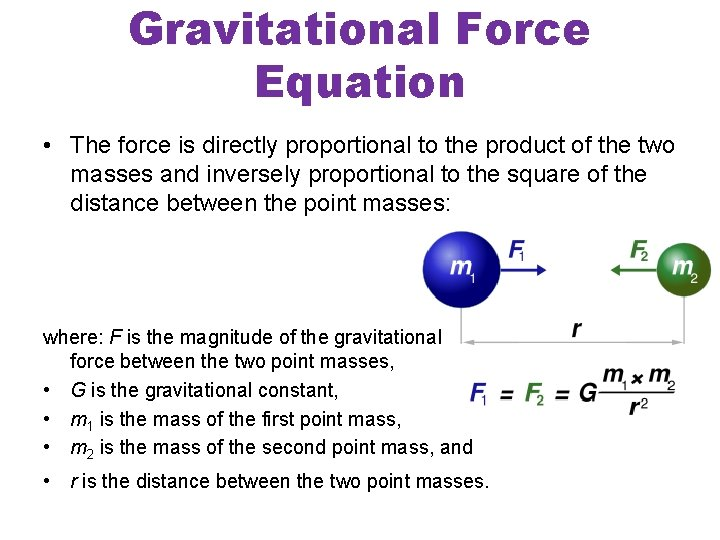Gravitational Force Equation • The force is directly proportional to the product of the two masses and inversely proportional to the square of the distance between the point masses: where: F is the magnitude of the gravitational force between the two point masses, • G is the gravitational constant, • m 1 is the mass of the first point mass, • m 2 is the mass of the second point mass, and • r is the distance between the two point masses.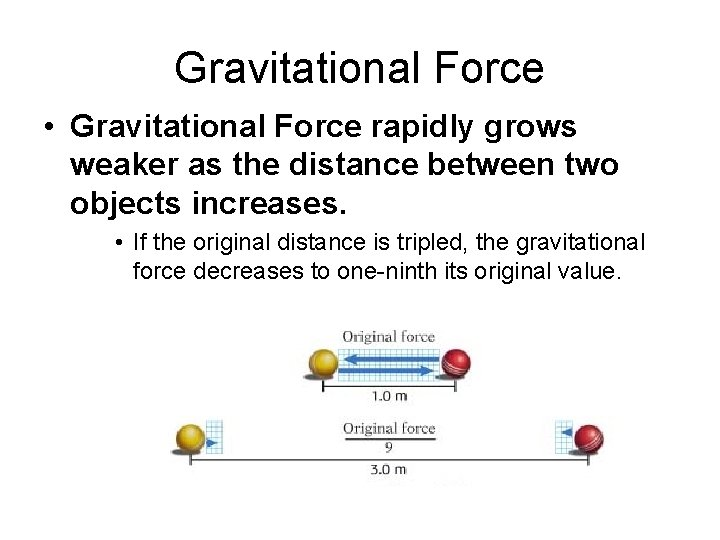Gravitational Force • Gravitational Force rapidly grows weaker as the distance between two objects increases. • If the original distance is tripled, the gravitational force decreases to one-ninth its original value.Gravitational Force • Gravitational force is weaker than other types of forces, even though it holds the planets, stars, and galaxies together.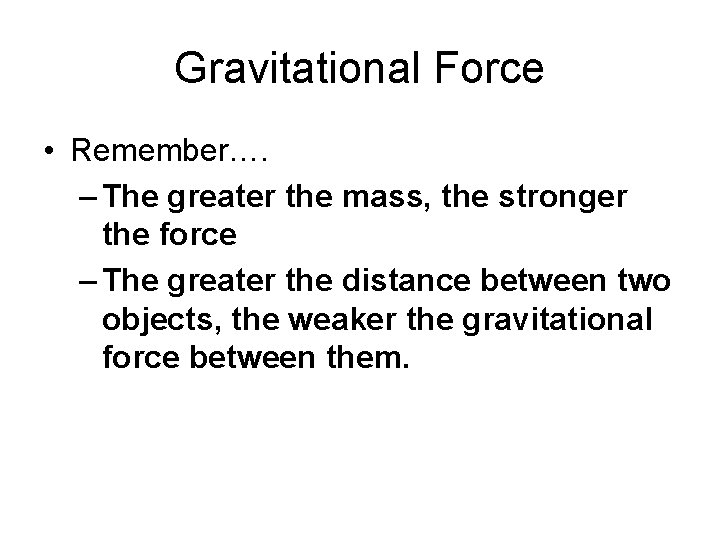Gravitational Force • Remember…. – The greater the mass, the stronger the force – The greater the distance between two objects, the weaker the gravitational force between them.• Introduction to Gravity - You. Tube. mp 4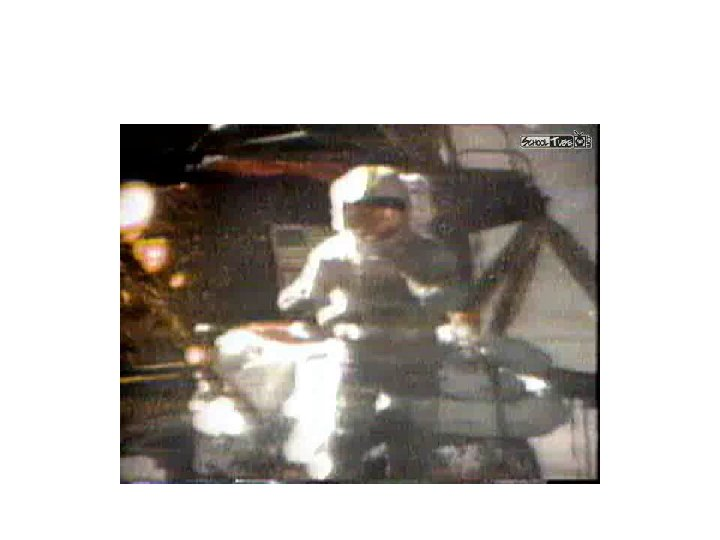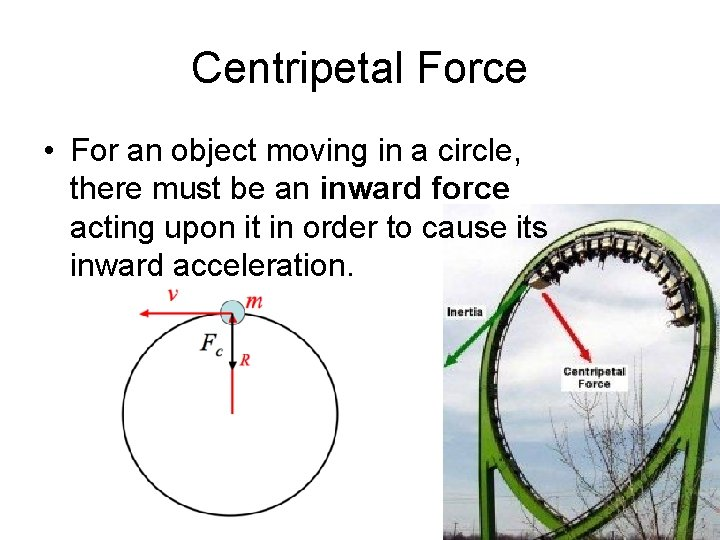Centripetal Force • For an object moving in a circle, there must be an inward force acting upon it in order to cause its inward acceleration.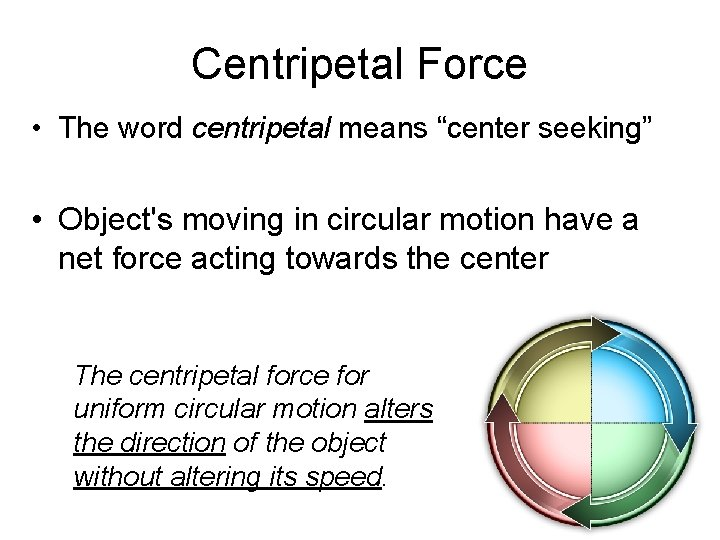Centripetal Force • The word centripetal means “center seeking” • Object's moving in circular motion have a net force acting towards the center The centripetal force for uniform circular motion alters the direction of the object without altering its speed.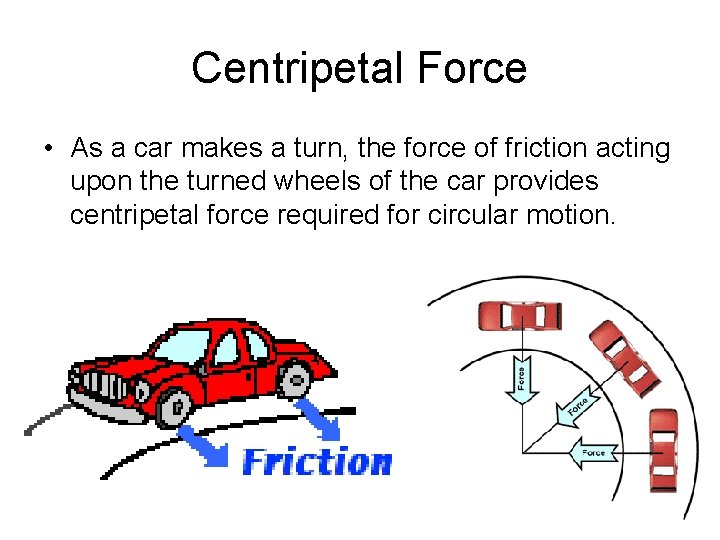Centripetal Force • As a car makes a turn, the force of friction acting upon the turned wheels of the car provides centripetal force required for circular motion.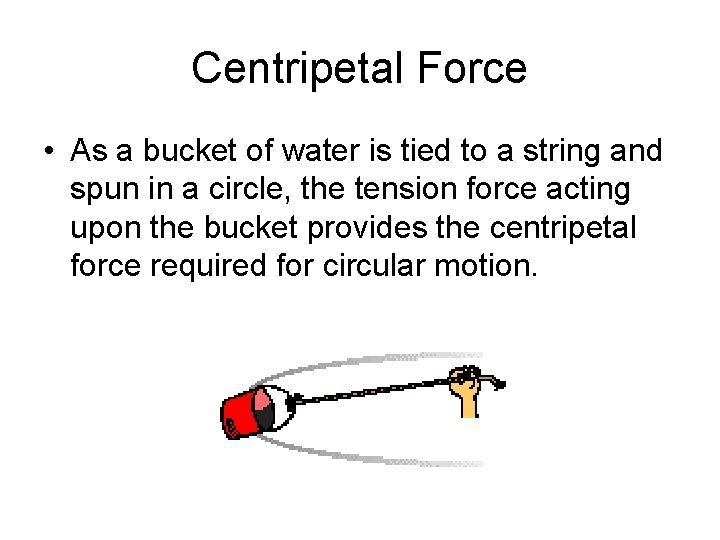Centripetal Force • As a bucket of water is tied to a string and spun in a circle, the tension force acting upon the bucket provides the centripetal force required for circular motion.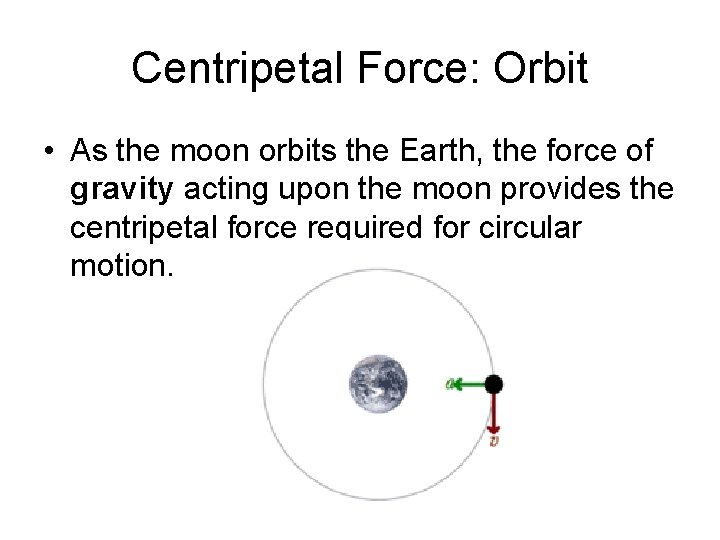Centripetal Force: Orbit • As the moon orbits the Earth, the force of gravity acting upon the moon provides the centripetal force required for circular motion.